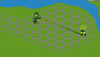# SOLVEDA* pathfinding issue

#### Geners

##### Member
So I have a grid of hexes, each containing a coordinate, an array of its neighbors, and its g_value, h_value, and f_value.

I have set up this A* pathfinding function to find a path between two points, and as you can see below it works perfectly if there's a valid path.But if there's not a valid path, instead of returning noone like I want it to it to, the loop runs forever and it keeps rechecking things that have already been evaluated, but that makes no sense because it shouldn't check anything that's contained in the closedSet

Here's my code for the pathfinding function. I'm sorry if this is very sloppy, it's my first attempt at this algorithm. As far as I can see, it's not an issue with any of my utility functions like "array_push" or with the hex objects themselves.

GML:
``````function hex_pathfinding(beginX, beginY, endX, endY){

var openSet = ds_list_create();
var closedSet = ds_list_create();
var current = global.hexGrid[beginX, beginY];
var beginning_hex = current;
var end_hex = global.hexGrid[endX, endY];

while (!ds_list_empty(openSet)) {

//shorthand
var setLength = ds_list_size(openSet);
var lowestValue = 0;
for(var i = 0; i < setLength; i++) {
if(openSet[| lowestValue].f_value > openSet[| i].f_value) {
lowestValue = i;
}
}

current = openSet[| lowestValue];
show_debug_message(string(current.coordinate) + ", " + string(current.coordinate));

if(current == end_hex) {
var path = [];
var tempH = current;
while(tempH != beginning_hex) {

path = array_push(path,tempH);
tempH = tempH.previousHex;

}
path = array_push(path,beginning_hex);
return path;
}

openSet = remove_from_ds(openSet, current);

//creating a shorthand
var neighbors = current.neighbors;

for(var i = 0; i < array_length(neighbors); i++) {

if(!contains_ds(closedSet,neighbors[i])) {

var tempG = current.g_value + 1;
if(contains_ds(openSet,neighbors[i])) {

if(tempG <= neighbors[i].g_value) {
neighbors[i].g_value = tempG;
neighbors[i].h_value = distance_hex(neighbors[i].coordinate,neighbors[i].coordinate,endX,endY);
neighbors[i].f_value = neighbors[i].g_value + neighbors[i].h_value;
neighbors[i].previousHex = current;
}
}
else {

neighbors[i].g_value = tempG;
neighbors[i].h_value = distance_hex(neighbors[i].coordinate,neighbors[i].coordinate,endX,endY);
neighbors[i].f_value = neighbors[i].g_value + neighbors[i].h_value;
neighbors[i].previousHex = current;

}

}

}

}

//this only happens when there is no valid path.
return noone;

}``````
So I guess my question is, what am I doing wrong?

Last edited:

#### Nidoking

##### Member
openSet = remove_from_ds(openSet, current);
For one thing, why are you storing nothing into the list variables?

For another thing, what are contains_ds and remove_from_ds?

•Geners

#### Geners

##### Member
For one thing, why are you storing nothing into the list variables?

For another thing, what are contains_ds and remove_from_ds?
What do you mean storing nothing into the list variables? I'm adding things into openSet when they need to be evaluated, then removing them from openSet and putting them in closedSet when they're done being evaluated.

remove_from_ds removes an index from that ds list that contains the value specified

GML:
``````//easy way to remove a given value from a ds list
function remove_from_ds(listID, value){
var listLength = ds_list_size(listID);
for(var i = listLength - 1; i >= 0; i--) {
if(listID[| i] == value) {
listID = ds_list_delete(listID,i);
return listID;
}
}

}``````

contains_ds, which I probably should have named ds_contains for readability, checks if any index in the list holds the value specified. If it does it returns true, if not it returns false.

GML:
``````//checks to see if a given value is contained in a given ds list
function contains_ds(listID, value){

for(var i = 0; i < ds_list_size(listID); i++) {
if(listID[| i] == value) {

return true;

}
}

return false;

}``````

#### TailBit

##### Member
GML:
``                listID = ds_list_delete(listID,i);``
ds_list_delete returns NA

so that's a problem

tho you could just use

remove_from_ds
Code:
``````var pos = ds_list_find_index(listID, value);
if pos != -1{
ds_list_delete(listID,pos)
return true;
}
return false;``````
but only do:
remove_from_ds(openSet, current);
as there is no reason to overwrite the list

contains_ds
Code:
``return ds_list_find_index(listID, value) != -1;``

•Geners

#### Nidoking

##### Member
What do you mean storing nothing into the list variables?
Well, normally, when you store something in a variable, it's a value of some kind. What you're storing here is nothing. It's not a value. It's not a thing. It's nothing. There is no "it" to be a thing because there's no thing. Observe:

listID = ds_list_delete(listID,i);
return listID;
What is the return value of ds_list_delete? Hint: It's the sound of one hand clapping.

•Geners

#### Geners

##### Member
What is the return value of ds_list_delete? Hint: It's the sound of one hand clapping.

GML:
``                listID = ds_list_delete(listID,i);``
ds_list_delete returns NA

Code:
``return ds_list_find_index(listID, value) != -1;``

Oh lord, I'm so used to functions that return an updated version of whatever you're parsing, I didn't even check the return values of these functions.

I feel like such a dumbass. You guys are great, thank you so much.

#### TailBit

##### Member
Yeah, seems it is the same with ds_list_add that Nidoking highlighed
GML:
``closedSet = ds_list_add(closedSet, current);``

•Geners

#### Geners

##### Member
Yeah, seems it is the same with ds_list_add that Nidoking highlighed
GML:
``closedSet = ds_list_add(closedSet, current);``
This explains why my array_push function was working fine but all of my ds_list data was failing. I'm shocked the pathfinding even worked.

#### Geners

##### Member
Okay, it works perfectly now. It both finds the optimal path and returns noone when a valid path isn't found. Thanks for all the help guys. I'm marking this as resolved.

•TailBit